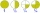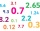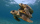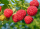# Difference of two fractions

What is the difference between 1/2 and 1/6?
(Write the answer as a fraction in lowest terms. )

x =  0.3333

### Step-by-step explanation:

$x=\frac{1}{2}-\frac{1}{6}=\frac{1}{3}=0.3333$Did you find an error or inaccuracy? Feel free to write us. Thank you!Tips to related online calculators
Need help to calculate sum, simplify or multiply fractions? Try our fraction calculator.

## Related math problems and questions:

• Circular gardenAlice creates a circular vegetable garden. Tomatoes are planted in 1/3 of the circular garden, carrots are planted in 2/5 of the circular garden, and green peppers are planted in 1/10 of the circular garden. What fraction represents the remaining unplante
• Evaluate - lowest termsEvaluate: 16/25 - 11/25 (Express answer as a fraction reduced to lowest terms. )
• Lowest terms 2The expression 4/12 can be expressed in its lowest term as 1/3. What is 3/15 expressed in its lowest term?
• Difference mixed fractionsWhat is the difference between 4 2/3 and 3 1/6?
• Evaluate 17Evaluate 2x+6y when x=- 4/5 and y=1/3. Write your answer as a fraction or mixed number in simplest form.
• Lowest termsReduce to the lowest terms: 32/124
• Divide 11Divide the product of 4 and 5/8 by 1 1/2. Write your answer as a mixed number.
• ShopperEva spent 1/4 in one store and 1/3 in another. What fraction is left?
• What is one thirdWhat is 1/3 as a decimal? Give your answer rounded to 2 decimal places.
• Fractions and mixed numerals(a) Convert the following mixed numbers to improper fractions. i. 3 5/8 ii. 7 7/6 (b) Convert the following improper fraction to a mixed number. i. 13/4 ii. 78/5 (c) Simplify these fractions to their lowest terms. i. 36/42 ii. 27/45 2. evaluate the follow
• Mixed numbersRewrite mixed numbers, so the fractions have the same denominator: 5 1/5 - 2 2/3
• A turtleA turtle is 20 5/6 inches below the surface of a pond. It dives to a depth of 32 1/4 inches. What is the change in the turtle’s position? Write your answer as a mixed number.
• Find the 24Find the difference between 2/7 and 1/21
• CarrieCarrie picked 2/5 of the raspberries from the garden, and Robin picked some too.  When they were finished, 1/3 of the raspberries still needed to be picked.  What fraction of the raspberries did Robin pick? Use pictures, numbers or words and write your fi
• Write 2Write 791 thousandths as fraction in expanded form.
• FractionsSort fractions z1 = (6)/(11); z2 = (10)/(21); z3 = (19)/(22) by its size. Result write as three serial numbers 1,2,3.
• Guess a fractionTom was asked to guess a fraction. The sum of 1/2 the numerator and 1/3 of its denominator is 30. If Tom subtracts 36 from its denominator, the fraction becomes 1/3. What is the fraction that Tom was asked to guess? (Leave your answer in simplest form)